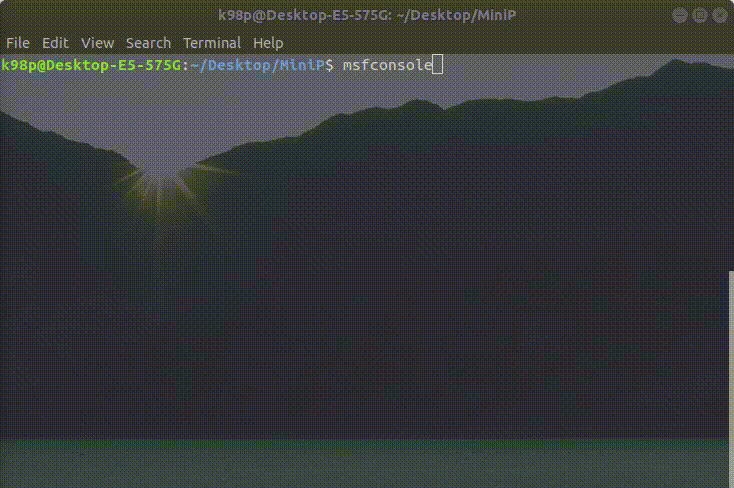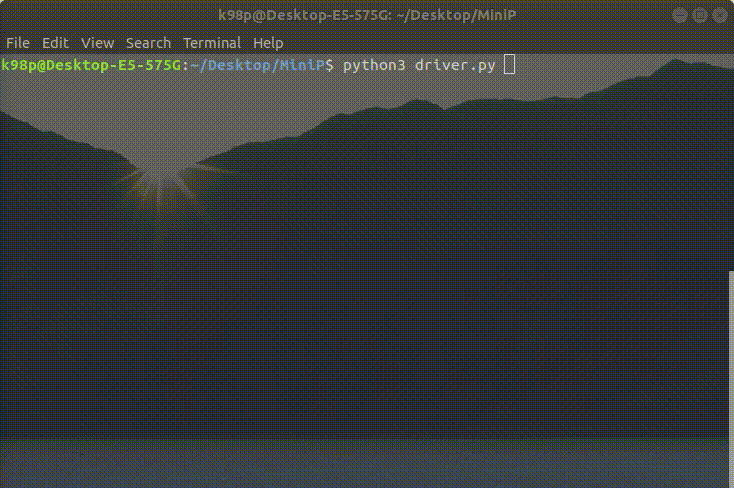# Python | Create simple animation for console-based application

As we know Python is a scripting language, and can be easily used to automate simple tasks. In this article, we will learn how to create a simple console-based animation, which can be used while developing a console based project as a utility.We will be using following modules –

```sys module
os module
time module
```

Below is the Python implementation:

 `# importing the necessary packages ` `import` `time ` `import` `sys ` `import` `os ` ` `  `# Function for implementing the loading animation ` `def` `load_animation(): ` ` `  `    ``# String to be displayed when the application is loading ` `    ``load_str ``=` `"starting your console application..."` `    ``ls_len ``=` `len``(load_str) ` ` `  ` `  `    ``# String for creating the rotating line ` `    ``animation ``=` `"|/-\\"` `    ``anicount ``=` `0` `     `  `    ``# used to keep the track of ` `    ``# the duration of animation ` `    ``counttime ``=` `0`         `     `  `    ``# pointer for travelling the loading string ` `    ``i ``=` `0`                      ` `  `    ``while` `(counttime !``=` `100``): ` `         `  `        ``# used to change the animation speed ` `        ``# smaller the value, faster will be the animation ` `        ``time.sleep(``0.075``)  ` `                             `  `        ``# converting the string to list ` `        ``# as string is immutable ` `        ``load_str_list ``=` `list``(load_str)  ` `         `  `        ``# x->obtaining the ASCII code ` `        ``x ``=` `ord``(load_str_list[i]) ` `         `  `        ``# y->for storing altered ASCII code ` `        ``y ``=` `0`                              ` `  `        ``# if the character is "." or " ", keep it unaltered ` `        ``# switch uppercase to lowercase and vice-versa  ` `        ``if` `x !``=` `32` `and` `x !``=` `46``:              ` `            ``if` `x>``90``: ` `                ``y ``=` `x``-``32` `            ``else``: ` `                ``y ``=` `x ``+` `32` `            ``load_str_list[i]``=` `chr``(y) ` `         `  `        ``# for storing the resultant string ` `        ``res ``=``''              ` `        ``for` `j ``in` `range``(ls_len): ` `            ``res ``=` `res ``+` `load_str_list[j] ` `             `  `        ``# displaying the resultant string ` `        ``sys.stdout.write(``"\r"``+``res ``+` `animation[anicount]) ` `        ``sys.stdout.flush() ` ` `  `        ``# Assigning loading string ` `        ``# to the resultant string ` `        ``load_str ``=` `res ` ` `  `         `  `        ``anicount ``=` `(anicount ``+` `1``)``%` `4` `        ``i ``=``(i ``+` `1``)``%` `ls_len ` `        ``counttime ``=` `counttime ``+` `1` `     `  `    ``# for windows OS ` `    ``if` `os.name ``=``=``"nt"``: ` `        ``os.system(``"cls"``) ` `         `  `    ``# for linux / Mac OS ` `    ``else``: ` `        ``os.system(``"clear"``) ` ` `  `# Driver program ` `if` `__name__ ``=``=` `'__main__'``:  ` `    ``load_animation() ` ` `  `    ``# Your desired code continues from here  ` `    ``# s = input("Enter your name: ") ` `    ``s ``=``"David"` `    ``sys.stdout.write(``"Hello "``+``str``(s)``+``"\n"``) `

Output:My Personal Notes arrow_drop_upCheck out this Author's contributed articles.

If you like GeeksforGeeks and would like to contribute, you can also write an article using contribute.geeksforgeeks.org or mail your article to contribute@geeksforgeeks.org. See your article appearing on the GeeksforGeeks main page and help other Geeks.

Please Improve this article if you find anything incorrect by clicking on the "Improve Article" button below.

Article Tags :

Be the First to upvote.

Please write to us at contribute@geeksforgeeks.org to report any issue with the above content.